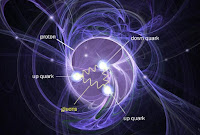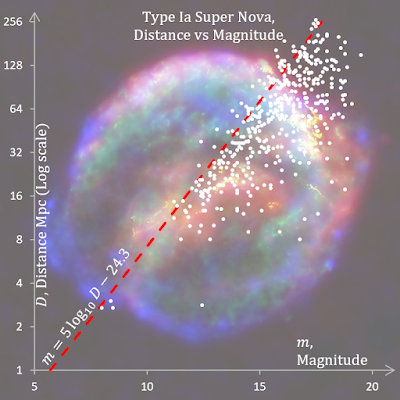## Friday, 16 April 2021

### Luminosity distance

In section 8.5 we are looking at redshifts and distances. We started in an FLRW universe with metric
\begin{align}{ds}^2=-{dt}^2+a^2\left(t\right)\left[\frac{{\rm dr}^2}{1-\kappa r^2}+r^2{d\theta}^2+r^2\sin^2{\theta}{d\phi}^2\right]&\phantom {10000}(1)\nonumber\end{align}or
\begin{align}{ds}^2=-{dt}^2+a^2\left(t\right){R_0}^2\left[{d\chi}^2+{S_k}^2\left(\chi\right){d\Omega}^2\right]&\phantom {10000}(2)\nonumber\end{align}where $k\in\left\{-1,0,1\right\}$ and
\begin{align}S_k\left(\chi\right)\ \equiv\left\{\begin{matrix}\sin{\chi},&k=+1&\rm{closed}\\\chi,&k=0&\rm{flat}\\\sinh{\chi}&k=-1&\rm{open}\\\end{matrix}\right.&\phantom {10000}(3)\nonumber\end{align}(2) is Carroll's 8.106, a sort of hybrid FLRW metric.

This starts at Carroll's 8.110 where he defines distance luminosity $d_L$ which you can get if you know the absolute luminosity of a star (or galaxy) and can measure the amount of light that reaches you. We then correct that for the expansion of the universe and further correct it because the universe deviates from a perfect sphere according to $S_k\left(\chi\right)$. We arrive at the celebrated and complicated formula at (4) which uses (5) which uses (6) .
\begin{align}d_L=\left(1+z\right)\frac{{H_0}^{-1}}{\sqrt{\left|\Omega_{c0}\right|}}S_k\left[\sqrt{\left|\Omega_{c0}\right|}\int_{0}^{z}\frac{dz^\prime}{E\left(z^\prime\right)}\right]&\phantom {10000}(4)\nonumber\end{align}where
\begin{align}E\left(z\right)=\left[\sum_{i(c)}{\Omega_{i0}\left(1+z\right)^{n_i}}\right]^\frac{1}{2}&\phantom {10000}(5)\nonumber\end{align}and there are four elements in the summation, one each for matter, radiation, curvature and vacuum energy density. $\Omega_i$ is the density parameter which we met before and was defined as
\begin{align}\Omega_i=\frac{8\pi G}{3H^2}\rho_i&\phantom {10000}(6)\nonumber\end{align}where the $\rho_i$ are the energy densities for each of those four things. $\Omega_i$ and $H$, the Hubble factor acquire a 0 subscript for their values in the present epoch.

I then try to calculate (4) from known values. $\Omega_{c0}$ the pseudo-density parameter for curvature comes from Friedmann's equation and the other three density parameters. That radiation energy is negligible, matter energy density corresponds to what is usually called ordinary (baryonic) plus dark matter and vacuum energy density is dark energy. The integral in (4) must be calculated numerically and I plotted below (page 10 in the pdf). After that we have plots of luminosity distance vs redshift for the three kinds of universe determined by $k$ in (3). Luminosity distances greater 100 billion light years are quite easy to get. I thought everything was a failure until I found some distance calculators which gave more or less the same! According to Carroll, these distances are compared with those measured from absolute and apparent luminosity. Luminosity distance is mighty peculiar. Coming soon: Proper motion distance and Angular diameter distance.

I also found that there is a missing divide by sign in Carroll's equation 8.122.
Read all about it at Commentary 8.5.2 Redshifts and Distances.pdf (12 pages)

## QuestionPoint particles in proton

For a system of discrete point particles the energy-momentum tensor takes the form$$T_{\mu\nu}=\sum_{a}{\frac{p_\mu^{\left(a\right)}p_\nu^{\left(a\right)}}{p^{0\left(a\right)}}\delta^{\left(3\right)}\left(\mathbf{x}-\mathbf{x}^{\left(a\right)}\right)}$$where the index $a$ labels the different particles. Show that, for a dense collection of particles with isotropically distributed velocities, we can smooth over the individual particle worldlines to obtain the perfect-fluid energy-momentum tensor 1.114.

1.114 was$$\mathbf{T}^{\mu\nu}=\left(\rho+p\right)U^\mu U^\nu+p\eta^{\mu\nu}$$where $\rho$ is the energy density of the fluid measured in its rest frame (rest-frame energy density) and $p$ is the isotropic rest-frame pressure. $T^{\mu\nu}$ is the energy-momentum tensor of a fluid element with a four velocity $U^\mu$.

Carroll is setting plenty of traps here.
• The $p$'s in second equation are rest-frame pressure and the $p$'s in the first are 4-momentum components.
• What does $\delta^{\left(3\right)}\left(\mathbf{x}-\mathbf{x}^{\left(a\right)}\right)$ mean? Carroll gives no hint.
• How do you smooth over the individual particle worldlines?
• Having indices down on the first equation is just perverse. Raise them immediately!
• There is a conceptual problem. The first equation concerns a point particle and the second concerns a particle of fluid which contains lots of point particles. I try to call the fluid particle a fluid element. It's a bit weird. We are doing summations over the point particles and we will be integrating and differentiating over fluid elements which are bigger but nevertheless still small enough to be treated as points in our calculus.
First I found out what $\delta^{\left(3\right)}\left(\mathbf{x}-\mathbf{x}^{\left(a\right)}\right)$ is and justified the expression for the energy-momentum of the point particle. I then got stuck until I found a solution by Alexey Bobrick on physics.stackexchange. It starts well but ends not so well, I believe. I think I did a little better.

## Saturday, 3 April 2021

### Super NovaKepler's Supernova* remnant in background

There is a unified catalogue of 5526 supernova (SN) by D. Lennarz et al and I want to extract the Type Ia Super Novae along with their magnitudes, redshifts and distances to see how those compare with what I have been learning out about redshifts and luminosity and other distances in section 8.3. Type Ia Super Nova are all supposed to have the same absolute magnitude of −19.3 (about 5 billion times brighter than the Sun). I was able to confirm Hubble's law and learnt that that the mighty peculiar formula for Type Ia Supernova: $m=5\log_{10}{D}-24.3$ for the fall off in apparent magnitude $m$ with distance $D$ is in fact an inverse power law. And that corrections for the expansion and curvature of the universe at this range (about a fifteenth of the size of the visible universe) are immaterial.